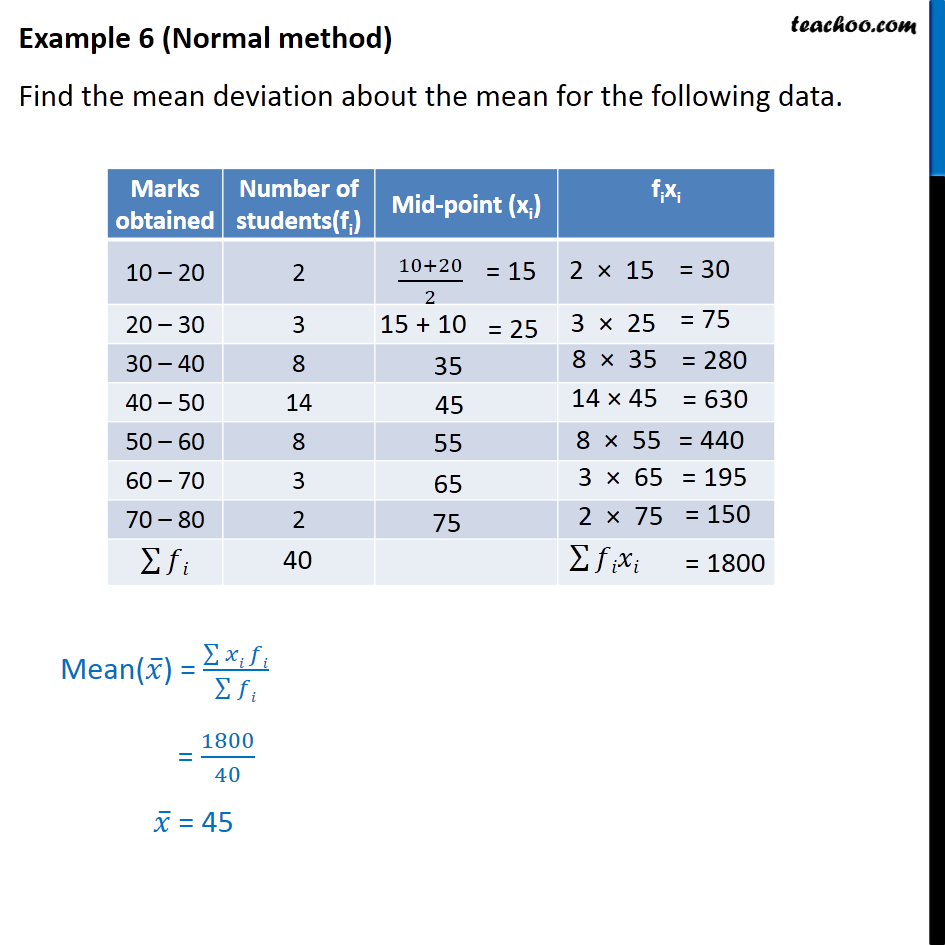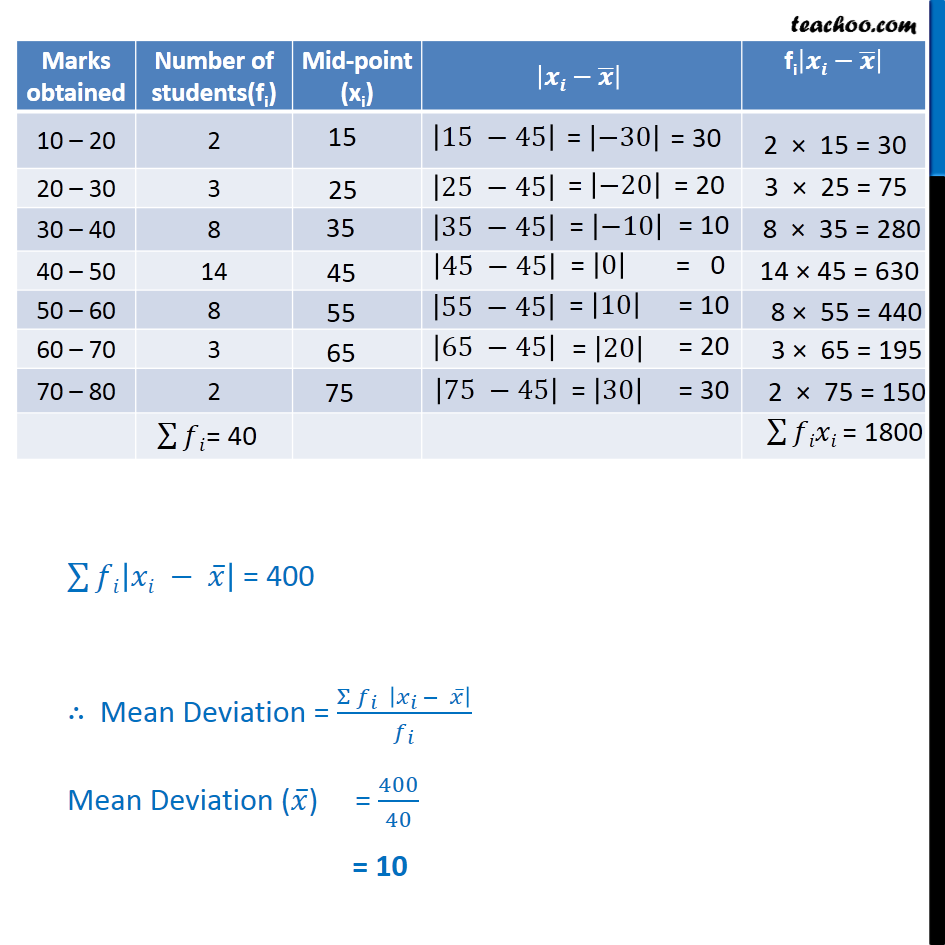Mean deviation about mean - Continuous frequency distibution

Chapter 13 Class 11 Statistics
Concept wiseLearn in your speed, with individual attention - Teachoo Maths 1-on-1 Class

### Transcript

Example 6 - Chapter 15 Class 11 Statistics - NCERT (Normal method) Find the mean deviation about the mean for the following data. Marks obtained Number of students(fi) Mid-point (xi) fixi 10 – 20 2 20 – 30 3 30 – 40 8 40 – 50 14 50 – 60 8 60 – 70 3 70 – 80 2 Mean(𝑥 ̅) = (∑▒〖𝑥𝑖 〗 𝑓𝑖)/(∑▒𝑓𝑖) = 1800/40 𝑥 ̅ = 45 ∑128▒𝑓𝑖|𝑥𝑖 − 𝑥 ̅ | = 400 ∴ Mean Deviation = (Σ 𝑓_𝑖 |𝑥_(𝑖 )− 𝑥 ̅ |)/𝑓_𝑖 Mean Deviation (𝑥 ̅) = 400/40 = 10